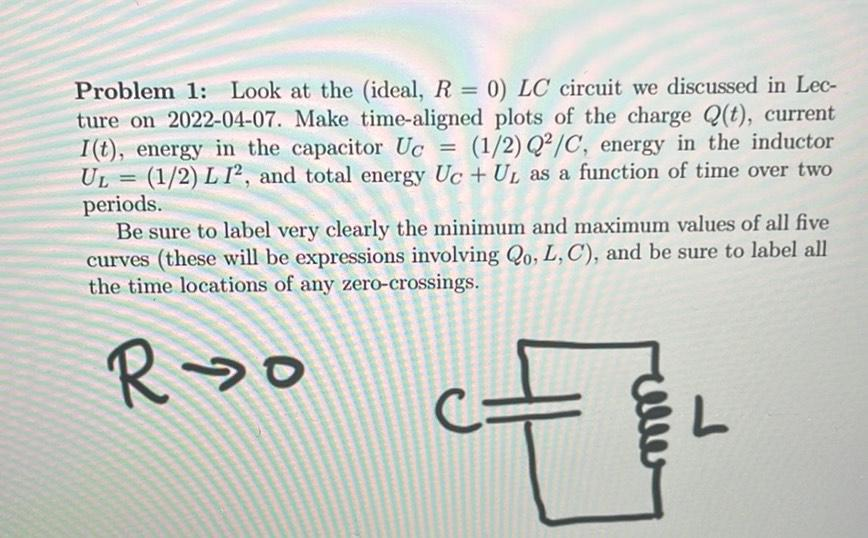Question:

# Problem 1: Look at the (ideal, R = 0) LC circuit we discussed in Lec- ture on 2022-04-07. Make time-aligned plots of the chargeProblem 1: Look at the (ideal, R = 0) LC circuit we discussed in Lec- ture on 2022-04-07. Make time-aligned plots of the charge Q(t), current I(t), energy in the capacitor Uc = (1/2)Q2/C, energy in the inductor UL = (1/2) L 12, and total energy Uc + U, as a function of time over two periods. Be sure to label very clearly the minimum and maximum values of all five curves (these will be expressions involving Qo, L,C), and be sure to label all the time locations of any zero-crossings. Ro o alle